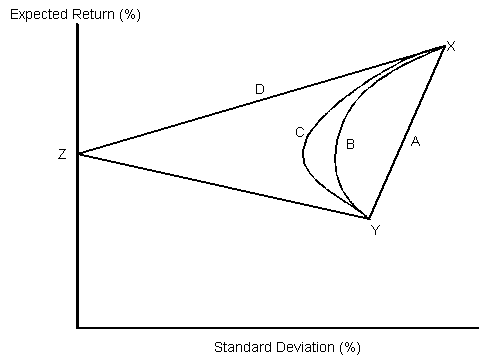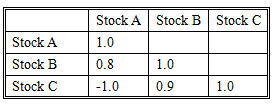### Seeing is believing!

Before you order, simply sign up for a free user account and in seconds you'll be experiencing the best in CFA exam preparation.

### Subject 3. Correlation and diversification

A diversification benefit is a reduction in portfolio standard deviation of return through diversification without an accompanying decrease in expected return. Portfolio diversification is affected by the number of assets in the portfolio and the correlation between these assets.

Correlation

The trade-off between risk and return for a portfolio depends not only on the expected asset returns and variances but also on the correlation of asset returns. The correlation between two assets represents the degree to which assets are related.

The correlation is the engine that drives the whole theory of portfolio diversification. The following figure illustrates minimum-variance frontier of a two-asset portfolio for four different correlations.The endpoints (X and Y) for all the frontiers are the same, since at each endpoint the expected return and standard deviation are simply the expected return and standard deviation of either asset.

• A: correlation = 1. This indicates a perfect linear relationship between the two assets. Diversification has no potential benefits.
• B: correlation = 0.5. Portfolio diversification can be achieved. The lower the correlation, the greater the diversification benefits.
• C: correlation = 0. This indicates there is no linear relationship between the two assets. More diversification can be achieved then B.
• D: correlation = -1. This indicates a perfect inverse linear relationship. Notice the minimum-variance frontier has two linear segments: XZ and ZY. XZ (line D) is the efficient frontier. The risk of the portfolio can be reduced to zero if desired.

The conclusion: As the correlation between two assets decreases, the diversification benefits increase.

Effect of Number of Assets on Portfolio Diversification

For an equally-weighted portfolio, its variance isσ2-bar is the average variance of return across all stocks, and Cov-bar is the average covariance of all pairs of two stocks.

Note that if n gets large enough:

• The first component becomes very small.
• The second component gets close to Cov-bar.

Therefore, the variance of an equally-weighted portfolio approximately equals the average covariance as the number of assets becomes large.

Example

Assume portfolio A has 2 assets and portfolio B has 30 assets. They are both equally weighted. The average asset variance is 0.5 and the average covariance is 0.3.

The variance of A is 1/2 0.5 + 1/2 0.3 = 0.4.
The variance of B is 1/30 0.5 + 29/30 0.3 = 0.31.

Portfolio B which has more assets has a lower variance.

In general, as the number of stocks increases, the variance of the portfolio will decrease.

• It takes less than 30 stocks to achieve 90% of the diversification benefit.
• The higher the average correlation, the greater the number of stocks needed to achieve a specified risk reduction.
• If you add more stocks to the portfolio, the standard deviation of the portfolio will eventually reach the level of the market portfolio.

#### Practice Question 1

The greatest portfolio diversification is achieved if the correlation between assets equals

A. -1.
B. 0.
C. 1.
D. - infinity.

Correlation is a number between -1 and +1. The lower the correlation, the bigger the diversification benefits.

#### Practice Question 2

In large portfolios, ______ is the most important factor to determine the portfolio risk.

A. Individual asset risks.
B. Average individual asset variance.
C. Average covariance.
D. Market risk.

The variance of a portfolio approaches the average covariance as the number of assets gets large.

#### Practice Question 3

It is NOT possible to construct a portfolio with zero variance of expected returns from assets whose expected returns have positive variance individually. True or false?

#### Practice Question 4

Adding another security to a portfolio of stocks will reduce the risk of the portfolio if the additional security

A. has returns that are negatively correlated with the other stocks in the portfolio.
B. is from an industry that is not already represented in the portfolio.
C. has returns that are positively correlated with the largest holdings in that portfolio.

#### Practice Question 5

Portfolio theory as described by Markowitz is most concerned with:

A. the identification of unsystematic risk.
B. the elimination of systematic risk.
C. the effect of diversification on portfolio risk.

#### Practice Question 6

The correlation coefficient of Portfolio X's returns and the market's returns is 0.95, and the correlation coefficient of Portfolio Y's returns and the market's returns is 0.40. Which of the following statements best describes the levels of portfolio diversification?

A. Both Portfolio X and Portfolio Y are poorly diversified.
B. Portfolio X is well diversified and Portfolio Y is poorly diversified.
C. Portfolio X is poorly diversified and Portfolio Y is well diversified.

#### Practice Question 7

Assume that a risk-averse investor owning stock in White Corporation decides to add the stock of either Black or Green Corporation to her portfolio. All three stocks offer the same expected return and total risk. The covariance of returns between White and Black is -0.05 and between White and Green is +0.05. Portfolio risk is expected to:

A. decline more by buying Black.
B. decline more by buying Green.
C. increase by buying either Black or Green.

#### Practice Question 8

If you desire maximum diversification, you should search for stocks with a correlation coefficient equal to:

A. +1.0.
B. 0.0.
C. -1.0.

#### Practice Question 9

Which statement is true?

I. The higher the average correlation, the fewer stocks we need to achieve a target risk reduction.
II. The variance of an equally-weighted portfolio approaches the average variance as n gets large.

I: The lower the correlation, the greater the diversification benefits.
II: It approaches the average covariance.

#### Practice Question 10

Stocks A, B, and C each have the same expected return and standard deviation. Given the correlation coefficients between these stocks shown in the table below, which combination of these stocks will result in the lowest risk portfolio?A. equally invested in stocks A and B.
B. equally invested in stocks B and C.
C. equally invested in stocks A and C.

Everything else being equal, the portfolio that has the lowest correlation, or most negative, will have the lowest risk.

#### Practice Question 11

To effectively diversify a portfolio an investor wants stocks with _________ correlation coefficients, the effect will be to ________ the standard deviation of the portfolio.

A. Negative; decrease
B. Negative; increase
C. Zero; increase

Diversification benefits: risk reduction through a diversification of investments. Investments that are negatively correlated or that have low positive correlation provide the best diversification benefits. Such benefits may be particularly evident in an internationally diversified portfolio.

#### Practice Question 12

For a two-stock portfolio, what would be the preferred correlation coefficient between the two stocks?

A. -1.00
B. 0.00
C. 1.00

#### Practice Question 13

Which statement about portfolio diversification is correct?

A. Diversification reduces the portfolio's expected return because diversification reduces a portfolio's systematic risk.
B. As more securities are added to a portfolio, total risk typically would be expected to fall at a decreasing rate.
C. The risk-reducing benefits of diversification do not occur meaningfully until at least 30 individual securities are included in the portfolio.

#### Practice Question 14

Security A has an expected return of 18% and a standard deviation of 40%. Securities B and C each have expected returns of 12% and standard deviations of 20%. If the correlation between rates of return for A and B is 0.35, and for A and C is 0.85, then investors holding only A:

A. who want to reduce risk would be better off adding B to their portfolios.
B. who want to reduce risk would be better off adding C to their portfolios.
C. would need to know the correlation between rates of return for B and C to determine which security offers the best opportunity for risk reduction.